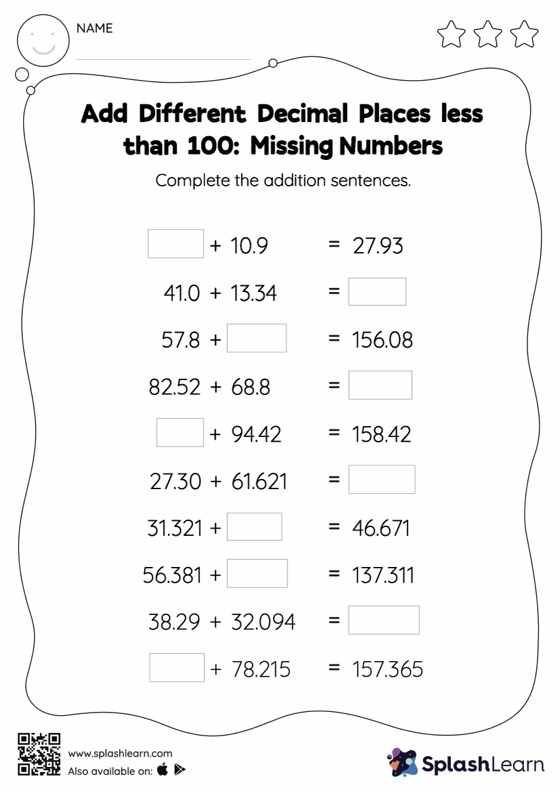# Add Different Decimal Places less than 100: Missing Numbers Worksheet

Home > Add Different Decimal Places less than 100: Missing NumbersChildren use their math skills to complete this add different decimal places less than 100 worksheet. Students align the decimal points and use zero as space holders to add decimals. Then they use the relationship between addition and subtraction to find the missing number in add different decimal places less than 100 worksheet. In each problem, the numbers are laid out in the horizontal format. Students should try to use different strategies involving composing and decomposing numbers to solve these problems. This will help them develop flexibility and fluency.